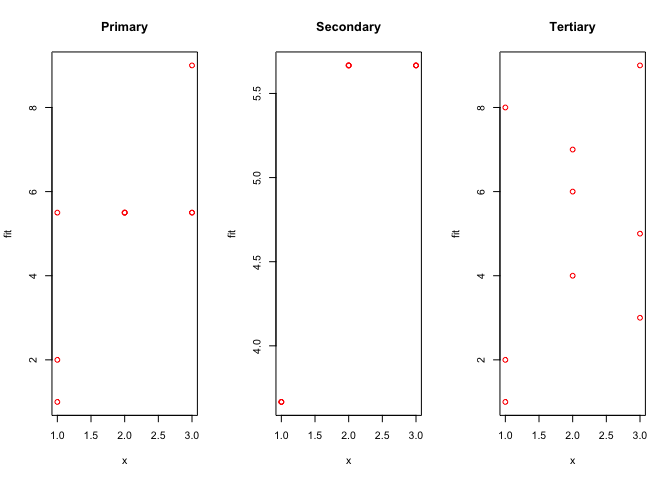Note: This is a working paper which will be expanded/updated frequently. The directory deleeuwpdx.net/pubfolders/isotone has a pdf copy of this article, the complete Rmd file that includes all code chunks, and R and FORTRAN files with the code. Suggestions are welcome 24/7.

# 1 Problem

In least squares monotone regression with a simple order we minimize a loss function of the form

$\begin{equation} \sigma(x)=\sum_{i=1}^n w_i(x_i-y_i)^2 \end{equation}$

over $$x_1\leq x_2\leq\cdots\leq x_n$$. The vector $$w$$ contains positive weights. The vector $$y$$ is the target. Monotone regression projects the target on the cone defined by the inequality constraints.

There are many R implementations available in various CRAN packages, starting with isoreg() in the stats package (if the weights are all equal). The function amalgm() in this paper is another such implementation, merely an R wrapper for a double precision modification of the FORTRAN code of Cran (1980). We could also have used the C implementation in recent versions of the smacof package. Various additions and improvements of the original package of De Leeuw and Mair (2009) are discussed in Mair, De Leeuw, and Groenen (2015).

If there are ties in the data the required order on $$x$$ needs to take those into account. There are three basic approaches. The first two are described by Kruskal (1964), the third is in De Leeuw (1977). In the primary approach. In the primary approach we require ordering only between tie blocks, not within tieblocks. The secondary approach requires equality within tie blocks and ordering between tie blocks, the tertiary approach only requires ordering of the tie block means.

So if the data used to generate the order are $\begin{bmatrix}2&2&3&1&3&3&1&2&1\end{bmatrix}$ then in the primary approach we require $\{x_4,x_7,x_9\}\leq\{x_1,x_2,x_8\}\leq\{x_3,x_5,x_6\},$ in the secondary approach this becomes $x_4=x_7=x_9\leq x_1=x_2=x_8\leq x_3=x_5=x_6,$ and in the tertiary approach it is $\frac{x_4+x_7+x_9}{3}\leq\frac{x_1+x_2+x_8}{3}\leq\frac{x_3+x_5+x_6}{3}$

# 2 Implementation

The code in the appendix implements the three approaches to ties by using a list of indices, computed by

f <- sort(unique(x))
g <- lapply(f, function (z) which(x == z))

Thus if the data are

##  2 1 3 2 1 3 3 1 2

then the list is

## []
##  2 5 8
##
## []
##  1 4 9
##
## []
##  3 6 7

The function isotone() uses simple list manipulations to get the data in the correct form for a call to amalgm(). Study it at your leisure.

# 3 Example

Again we use the same data to generate the order

##  2 1 3 2 1 3 3 1 2

We also choose the target

##  7 1 5 6 2 9 3 8 4

The primary approach gives

##  5.5 1.0 5.5 5.5 2.0 9.0 5.5 5.5 5.5

with a loss function value of 17.5. For the secondary approach the projection is

##  5.666667 3.666667 5.666667 5.666667 3.666667 5.666667 5.666667 3.666667
##  5.666667

with a loss function value of 52. And for the tertiary approach we find

##  7 1 5 6 2 9 3 8 4
with a loss function value of only 0. We show the transformations of the data in figure 1.Figure 1: Three approaches to ties

Note that in the tertiary aproach the transformation of the data is generally not monotone.

# 4 Appendix: Code

amalgm <- function (x, w = rep (1, length (x))) {
n <- length (x)
a <- rep (0, n)
b <- rep (0, n)
y <- rep (0, n)
lf <- .Fortran ("AMALGM", n = as.integer (n), x = as.double (x), w = as.double (w), a = as.double (a), b = as.double (b), y = as.double (y), tol = as.double(1e-15), ifault = as.integer(0))
return (lf\$y)
}

isotone <-
function (x,
y,
w = rep (1, length (x)),
ties = "secondary") {
f <- sort(unique(x))
g <- lapply(f, function (z)
which(x == z))
n <- length (x)
k <- length (f)
if (ties == "secondary") {
w <- sapply (g, length)
h <- lapply (g, function (x)
y[x])
m <- sapply (h, sum) / w
r <- amalgm (m, w)
s <- rep (0, n)
for (i in 1:k)
s[g[[i]]] <- r[i]
}
if (ties == "primary") {
h <- lapply (g, function (x)
y[x])
m <- rep (0, n)
for (i in 1:k) {
ii <- order (h[[i]])
g[[i]] <- g[[i]][ii]
h[[i]] <- h[[i]][ii]
}
m <- unlist (h)
r <- amalgm (m, w)
s <- r[order (unlist (g))]
}
if (ties == "tertiary") {
w <- sapply (g, length)
h <- lapply (g, function (x)
y[x])
m <- sapply (h, sum) / w
r <- amalgm (m, w)
s <- rep (0, n)
for (i in 1:k)
s[g[[i]]] <- y[g[[i]]] + (r[i] - m[i])
}
return (s)
}

# 5 NEWS

001 01/19/16 First release